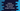# Use python bin() function to convert integer to binary## Use python bin() function to convert integer to binary:

For converting one integer to binary, we can write our own function that can take one integer value and return its binary equivalent. Or, we can use bin library function that does the same thing and we don’t need any other library for that.

In this post, I will show you how bin works with one example.

### Syntax of bin() :

The syntax of bin() is as below:

``bin(no)``

Here, we are passing one number no to this function. This number no is the number for which we are finding the binary value. It returns the binary representation in string format.

### Example of bin() :

Below is an example that uses bin to find the binary of different numbers :

``````print("Binary representation of 45 is ",bin(45))
print("Binary representation of 40 is ",bin(40))
print("Binary representation of 32 is ",bin(32))
print("Binary representation of 10 is ",bin(10))
print("Binary representation of 0 is ",bin(0))
print("Binary representation of 100 is ",bin(100))``````

It will print :

``````Binary representation of 45 is  0b101101
Binary representation of 40 is  0b101000
Binary representation of 32 is  0b100000
Binary representation of 10 is  0b1010
Binary representation of 0 is  0b0
Binary representation of 100 is  0b1100100``````

### Using bin with a custom class:

We can also use bin() with a custom class. For that, we need to implement index() method in that class that will return one integer value.

For example:

``````class Student:
def __init__(self, age, marks):
self.age = age
self.marks = marks

def __index__(self):
return self.age + self.marks

student = Student(5, 5)
print("Binary representation of Student(5,5) : ", bin(student))``````

It will print:

``Binary representation of Student(5,5) :  0b1010``

Here, index is returning the sum of age and marks in the Student class. For the Student object we created, it will return 10. So, when we are calling bin on the Student object, i.e. on student, it calculates the binary of 10 which is 0b1010.

This example shows you how to use bin to quickly find out the binary representation of a number in python. You can write your own function to do that or use bin for a quick alternative.